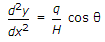# Civil Engineering - UPSC Civil Service Exam Questions

31.

In a particular material, if the modulus of rigidity is equal to the bulk modulus, then the Poisson's ratio will be

 A.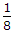B.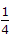C.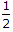D. 1

Explanation:

No answer description available for this question. Let us discuss.

32.

The weight of aggregate having specific gravity 2.65, completely filled into a cylinder of volume 0.003 m3 is 5.2 kg. What is the value of the angularity index of aggregate (approximately) as given by Murdock ?

 A. 1 B. 0.34 C. 0.15 D. 0.05

Explanation:

No answer description available for this question. Let us discuss.

33.

Which of the following pairs in respect of ordinary Portland cement (OPC) are correctly matched
1. Initial setting time....30 minutes
2. Final setting time....10 hours
3. Normal consistency....10%
Select the correct answer from the codes given below :

 A. 1, 2 and 3 B. 2 and 3 C. 1 and 2 D. 1 and 3

Explanation:

No answer description available for this question. Let us discuss.

34.

A spillway of an irrigation project is to be studied by means of a model constructed to a scale of 1 : 9. The prototype discharge is 1000 m3/s. Neglecting the viscous and surface tension effects, the required flow rate for the model is

 A. 12.35 m3/s B. 111.11 m3/s C. 4.11 m3/s D. 1.37 m3/s

Explanation:

No answer description available for this question. Let us discuss.

35.

Which one of the following is the correct differential equation of the shape of the cable (similar to electrical cables) of unit weight 'q' with small slopes, as shown in the given figure ?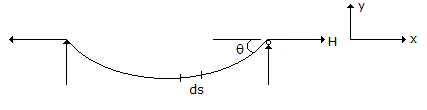A.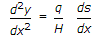B.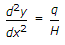C.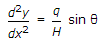D.